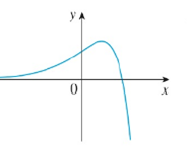Chapter 2, Problem 42RE### Single Variable Calculus: Early Tr...

8th Edition
James Stewart
ISBN: 9781305270343

#### Solutions

Chapter
Section### Single Variable Calculus: Early Tr...

8th Edition
James Stewart
ISBN: 9781305270343
Textbook Problem

# Trace or copy the graph of the function. Then sketch a graph of its derivative directly beneath.To determine

To sketch: The graph of derivative of f below the graph of f.

Explanation

From the given graph, it is observed that the graph of f contains a horizontal tangent at one point. Let this point be A.

Note that, the value of the derivative will be zero at the point where the function has the horizontal tangent.

Thus, the graph of f will be zero at the point A.

From the point A to left, the slope of the graph f is strictly positive which implies that the derivative graph f must have a functional value in positive

### Still sussing out bartleby?

Check out a sample textbook solution.

See a sample solution

#### The Solution to Your Study Problems

Bartleby provides explanations to thousands of textbook problems written by our experts, many with advanced degrees!

Get Started

#### In Exercises 6372, evaluate the expression. 64. 4 + |4|

Applied Calculus for the Managerial, Life, and Social Sciences: A Brief Approach

#### In problems 63-73, factor each expression completely. 70.

Mathematical Applications for the Management, Life, and Social Sciences

#### converges to: 2 the series diverges

Study Guide for Stewart's Multivariable Calculus, 8th

#### is the binomial series for:

Study Guide for Stewart's Single Variable Calculus: Early Transcendentals, 8th